DraftKings 2019 SEC Odds
-230
0.43 to 1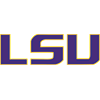LSU
59.7% implied probability

+175
1.75 to 1Georgia
31.2% implied probability

+2200
22 to 1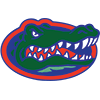Florida
3.7% implied probability

+4000
40 to 1Missouri
2.1% implied probability

No changes have been recorded yet.
+4000
40 to 1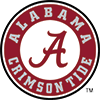Alabama
2.1% implied probability

+25000
250 to 1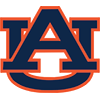Auburn
0.3% implied probability

+50000
500 to 1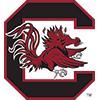South Carolina
0.2% implied probability

+50000
500 to 1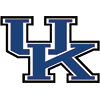Kentucky
0.2% implied probability

+50000
500 to 1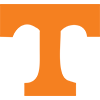Tennessee
0.2% implied probability

+50000
500 to 1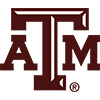Texas A&M
0.2% implied probability

+100000
1000 to 1Vanderbilt
0.1% implied probability

+100000
1000 to 1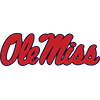Mississippi
0.1% implied probability